# Fun with Fractals¶

The most famous fractal equation is the Mandelbrot set:

$$z_{i+1} = z_i^2 + z_0$$

But what is actually going on with this function? How does this generate all the fun colors and designs that you see in fractal images? How do those fractal artists come up with images that look like 3D objects? Can you do all this in Python? This guide started as a Python notebook I used to explore the equations and help me to understand what is actually happening when these images are generated.

In addition to Python and the Jupyter notebook, this guide requires only matplotlib and numpy. For saving the images to a file, we'll use the Pillow package.

In :
import numpy as np
import matplotlib.pyplot as plt
from matplotlib.patches import Circle
%matplotlib inline
np.seterr(over='ignore', invalid='ignore');  # Ignore floating point overflows


## Complex Numbers¶

Fractals are generated by iterative functions that operate on complex numbers. The $z$ variables in the equation above are complex numbers. Don't worry, they're not all that complex. Think of them as two numbers lumped together into a single variable. These two numbers are called the real part and the imaginary part. In this application, think of a complex number just defining a point in a 2D plane, with the real part as the x-coordinate and the imaginary part as the y-coordinate.

In Python, complex numbers can be entered directly using a "j" after the imaginary part. All the math operations are handled automatically.

In :
c = .75 + 0.5j  # This is a complex number in Python.
plt.plot(c.real, c.imag, marker='o')   # The two componets, 0.75 and 0.5, are accessed using .real and .imag.
plt.xlim(-1,1)
plt.ylim(-1,1)
plt.gca().set_aspect('equal')
plt.axhline(0, ls=':', color='black')
plt.axvline(0, ls=':', color='black')
plt.xlabel('Real Part')
plt.ylabel('Imaginary Part');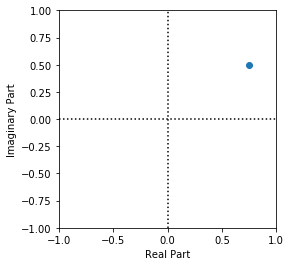To make a numpy array using complex numbers, either pass in complex Python objects or use the 'complex' parameter

In :
np.zeros(4, 'complex')

Out:
array([ 0.+0.j,  0.+0.j,  0.+0.j,  0.+0.j])

## Recurrence Equations and Iterations¶

Now we're ready to explore the Mandelbrot equation $z_{i+1} = z_i^2 + z_0$. Here $z_0$ is the coordinate of each pixel in the image. Starting with this $z_0$, we calculate $z_1$, $z_2$, and so on until we decide to stop. To help see what's going on, use the following function. It takes a single complex number, or point in the image, and runs it through the equation a specific number of times, plotting how the point moves around with each iteration.

In :
def plot_mandelpoint(z, iters, escape=2):
''' Trace a single point z through Mandelbrot iterations '''
z0 = z                     # Save off initial point
zlast = z               # Keep track of last point so we can draw arrows

plt.figure(figsize=(5,5))
plt.plot(z.real, z.imag, marker='x', color='C0')  # Initial point
for i in range(iters):
z = z*z + z0           # The actual Mandelbrot equation.

plt.plot(z.real, z.imag, marker='x', color='C0')
if zlast is not None:
plt.arrow(zlast.real, zlast.imag,
dx=(z.real-zlast.real), dy=(z.imag-zlast.imag),
zlast = z
if abs(z) > escape:
print('Escaped in {} iterations'.format(i+1))
break

plt.axhline(0, ls=':', color='black')
plt.axvline(0, ls=':', color='black')
plt.xlim(-escape-.1, escape+.1)
plt.ylim(-escape-.1, escape+.1)


Use the plot_mandelpoint function with iters=0 to just plot a point in the image. Here we'll choose $z_0 = .4+.4j$.

In :
z0 = .4+.4j   # Plot only z0, one initial starting point
plot_mandelpoint(z0, iters=0)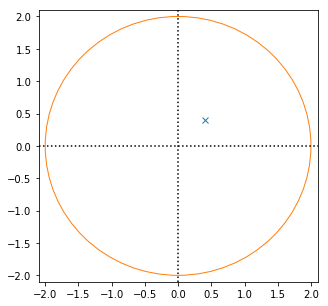Now the point goes throught the recurrence equation:

$z_1 = z_0^2 + z_0 = (.4 + .4j)(.4 + .4j) + .4+.4j = 0.4 + 0.72j$

In :
plot_mandelpoint(z0, iters=1)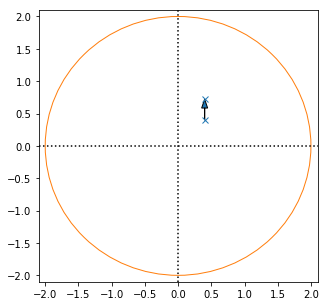The point moves a bit.

Keep going and calculate $z_2 = z_1^2 + z_0$...

In :
plot_mandelpoint(z0, iters=2)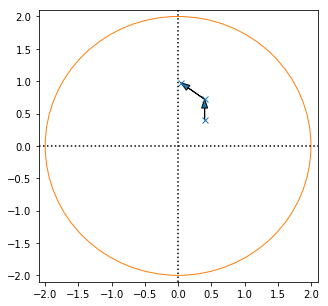A few more times... the point moves each iteration. This is called the point's orbit.

In :
plot_mandelpoint(z0, iters=6)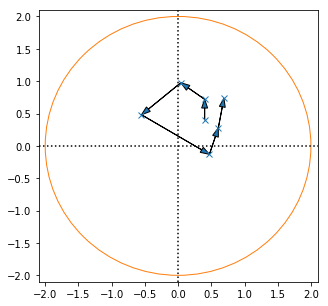If we keep orbiting, the point may keep going in circles or it may eventaully "escape" to infinity. I don't have time to calculate until infinity, so instead, we can set some threshold and assume it will escape to infinity if the point exceeds the threshold. Typically, a good choice is $R=2$ shown by the orange circle.

This point escaped after 8 iterations.

In :
plot_mandelpoint(z0, iters=8)

Escaped in 8 iterations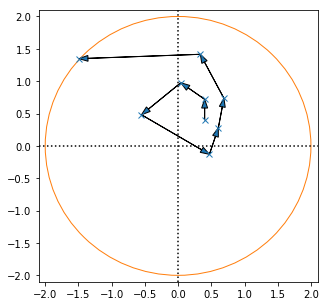What we need now is a way to translate the 7 iterations into a color for the pixel at this coordinate. Using matplotlib's colormap is an easy way to do this. The imshow() function automatically normalizes the value and looks up the corresponding color in the table.

In :
cmap = plt.get_cmap('magma')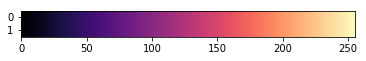Now we can determine the color of the one pixel, at point $z_0$ that we started with. Let's plot it (using a manual color lookup since we're not using imshow yet).

In :
plt.figure(figsize=(5,5))
plt.plot(z0.real, z0.imag, color=cmap(7/15), marker='.')
plt.axhline(0, ls=':', color='black')
plt.axvline(0, ls=':', color='black')
plt.xlim(-2.1, 2.1)
plt.ylim(-2.1, 2.1)
plt.gca().set_aspect('equal', 'datalim')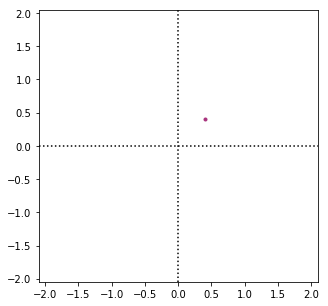Repeat this process for every pixel in the image. One pixel down, a few million to go...

Now try another $z_0$ coordinate in the image.

In :
plot_mandelpoint(-.5-.6j, iters=10)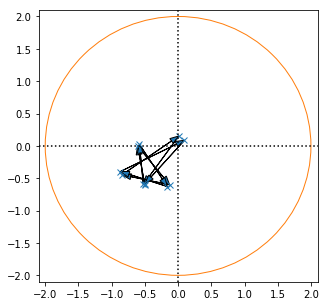This point will orbit around forever, and never get out of the circle! You can try increasing the iters parameter to demonstrate. We can't loop forever, so we'll set some threshold, maybe 100, and assume that if it hasn't escaped in 100 iterations, it's not going to. This point is considered to be "in the set." In this case, the pixel is colored with the lowest colormap value, or sometimes it is left black.

Now we have 2 pixels done! Here's what the image looks like so far:

In :
plt.figure(figsize=(5,5))
z01 = -.5-.6j
plt.plot(z01.real, z01.imag, color=cmap(0.0), marker='.')
z0 = .4+.4j
plt.plot(z0.real, z0.imag, color=cmap(7/15), marker='.')
plt.axhline(0, ls=':', color='black')
plt.axvline(0, ls=':', color='black')
plt.xlim(-2.1, 2.1)
plt.ylim(-2.1, 2.1)
plt.gca().set_aspect('equal', 'datalim')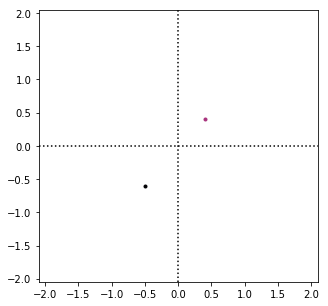#### TRY THIS¶

These are some ideas for exploring the data:

• Use other coordinates in the plot_mandelpoint() function. Try to find some that escape quickly, some that escape after a long time, and some that never escape.
• On points that never escape, try increasing the maximum iterations before giving up. Are they still stuck?

## All together now¶

In theory, you could now calculate the orbit of every point in an image and determine its color by hand. Obviously this would take millions of calculations on complex numbers for one image, way too much for hand-calculation, which is why fractals are considered computer-generated art.

When writing computer code to calculate all the pixels for you, looping through every pixel one at a time like we did above every iteration would be woefully inefficient. The next function vectorizes the calculation so all pixels in the image can be calculated at once.

In :
def mandelbrot(xrng, yrng, escape=2, maxiter=50, cmap='magma'):
''' Generate Mandelbrot set.

Parameters
----------
xrng: array of real values defining width of plot
yrng: array of imag values defining height of plot
escape: escape threshold
maxiter: maximum number of iterations before giving up
cmap: name of matplotlib colormap

The image size in pixels will be len(xrng) x len(yrng).
'''
z0 = xrng + 1.0j * yrng.reshape(-1, 1)
z = np.zeros(z0.shape, dtype='complex')
image = np.zeros((len(xrng), len(yrng)), dtype='int')  # The image data, how long each point takes to escape
mask = np.full(image.shape, True)   # True/False mask indicating whether each pixel has escaped yet

for i in range(maxiter):
# Calculate the Mandelbrot recurrence function, but only points that haven't escaped yet.
mask = abs(z) < escape  # Find points that still haven't escaped
image += mask           # And increase the iters of those points

# Plot it.
plt.figure(figsize=(8,8))
plt.imshow(image, aspect='equal', cmap=cmap, origin='lower',
extent=(xrng, xrng[-1], yrng, yrng[-1]))
return image


Now the entire mandelbrot set can be generated. Here it's 1000 x 1000 pixels. It's doing exactly the same thing we did above, taking each point $z_0$ and "orbiting" it around until it either escapes or we hit our maximum iteration limit, then setting the color based on how long it took to escape.

In :
image = mandelbrot(xrng=np.linspace(-2.4,1.2,1000), yrng=np.linspace(-1.4,1.4,1000), maxiter=50)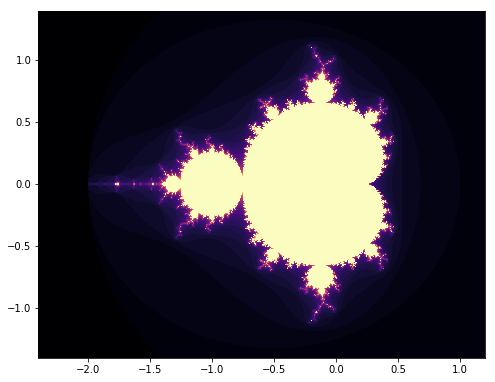Adjust the xrng and yrng linspace parameters to zoom in and move around.

In :
image = mandelbrot(xrng=np.linspace(-0.5,0,1000), yrng=np.linspace(-1.0,-0.5,1000), maxiter=100)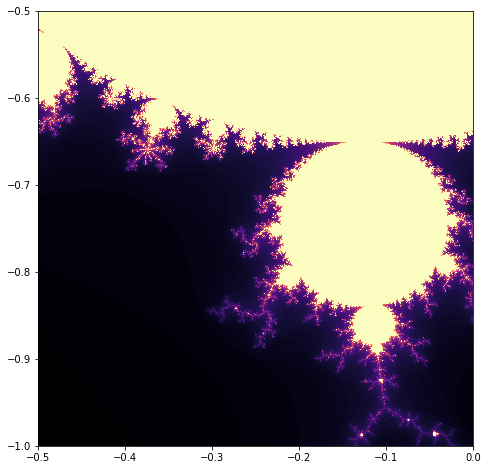#### TRY THIS:¶

• Try changing the maximum iterations, escape threshold, and colormap (see https://matplotlib.org/tutorials/colors/colormaps.html) to see what happens.

• Take some of the points you ran through plot_mandelpoint() and plot them on top of the full mandelbrot image. Use something like plt.plot(0.4, 0.4, marker='o', color='red')

• What happens when maxiter is very low, like 1 or 2?

• Create a Julia set function. Instead of iterating $z_{i+1} = z_i^2 + z_0$ (where $z_0$ is the initial value), use the recurrence function $z_{i+1} = z_i^2 + c$, where $c$ is any constant complex number. Experiment with different $c$ values.

• Try other recurrence functions. $z_i^4 + z_0$ is a good example.

## Orbit Traps¶

So far we've set each pixel's color according to how long it takes for its orbit to "escape". There are other ways to specify the color of a pixel going through the orbit while still using the same recurrence equation. For example, a if point falls within a specified region, say a circle or a band around the real axis, it is said to be "trapped" and the color is based on how far the point is from the center of that region.

This example defines a trap along the imaginary axis (re=0). If the point falls within 0.2 units of the axis, it falls in the trap and is colored based on how far it falls from the re=0 axis. Points that are not trapped within maxiter iterations remain black. This function shows a single point going through it's orbit, and whether it falls in the trap on each iteration.

In :
def plot_mandelorbit(z, iters, twidth=.1):
''' Trace a single point z through Mandelbrot iterations '''
z0 = z                     # Save off initial point
zlast = None               # Keep track of last point so we can draw arrows

plt.figure(figsize=(5,5))
for i in range(iters+1):
z = z*z + z0

if abs(z.real) < twidth/2:
print('Trapped in iteration {}, distance: {:.3f}'.format(i, abs(z.real)))
color = 'red'
marker = 'o'
else:
color = 'C0'
marker = 'x'
plt.plot(z.real, z.imag, marker=marker, color=color)
if zlast is not None:
plt.arrow(zlast.real, zlast.imag,
dx=(z.real-zlast.real), dy=(z.imag-zlast.imag),
zlast = z

plt.axhline(0, ls=':', color='black')
plt.axvline(0, ls=':', color='black')
plt.axvline(twidth/2, color='C1')
plt.axvline(-twidth/2, color='C1')
plt.xlim(-.8, .8)
plt.ylim(-.8, .8)
plt.gca().set_aspect('equal', 'datalim')

In :
plot_mandelorbit(.22+.41j, iters=5)

Trapped in iteration 2, distance: 0.045
Trapped in iteration 5, distance: 0.040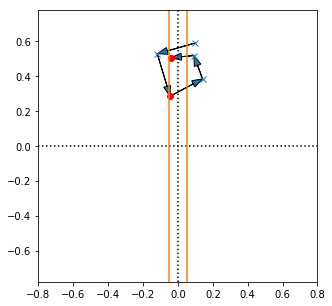The orage lines show the bounds of the trap. As the point moves through its orbit, the red points fall inside the trap. Notice that a point may fall in the trap multiple times as it orbits.

For a line trap like this one, the color can be based on distance between the point and the center of the trap. By fading from, say, bright blue if the point hits the center of the trap, to black if the point hits the very edge of the trap, a 3D look can be generated.

In case a point hits a trap multiple times, there are several options. The most common, and what we'll do here, is to set the color based on the first time the point hits the trap. You could also use the last time, the largest distance of all trap events, smallest distance, etc.

In :
def mandelbrottrap(xrng, yrng, trapwidth=.2, maxiter=10, cmap='Blues_r'):
''' Generate Mandelbrot set.

Parameters
----------
xrng: array of real values defining width of plot
yrng: array of imag values defining height of plot
trapwidth: width of trap about real axis
maxiter: maximum number of iterations before giving up
cmap: name of matplotlib colormap
'''
z0 = xrng + 1.0j * yrng.reshape(-1, 1)
z = np.zeros(z0.shape, dtype='complex')
image = np.zeros((len(xrng), len(yrng)))

for i in range(maxiter):
# Because traps can get hit multiple times, calculate all points every iteration (no masking).
z = z**2 + z0
# Find points that are within trapwidth of imaginary axis
# Fill in the image with the distance from axis

plt.figure(figsize=(8,8))
plt.imshow(image/trapwidth*2, aspect='equal', cmap=cmap, origin='lower',
extent=(xrng, xrng[-1], yrng, yrng[-1]))
return image


To better illustrate the trap, let's first run the function without any orbiting (by setting maxiter=1). This shows us what the trap looks like originally. As you can see, the trap looks like a cylinder at x=0.

In :
image = mandelbrottrap(np.linspace(-2.4,1.2,1000), np.linspace(-1.4,1.4,1000), maxiter=1)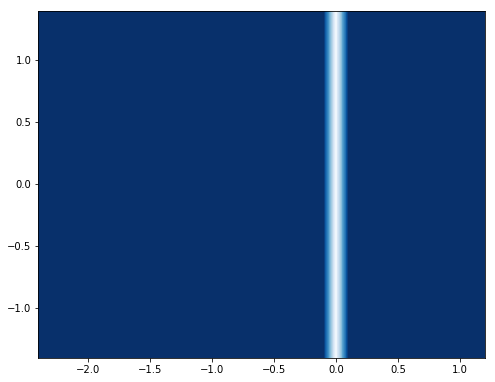Now allow the points to orbit by increasing the maxiter parameter. Try setting the maxiter value to numbers between 1 and 10 to see how "tubes" are added as iterations increase.

In :
image = mandelbrottrap(np.linspace(-2.4,1.2,1000), np.linspace(-1.4,1.4,1000), maxiter=10)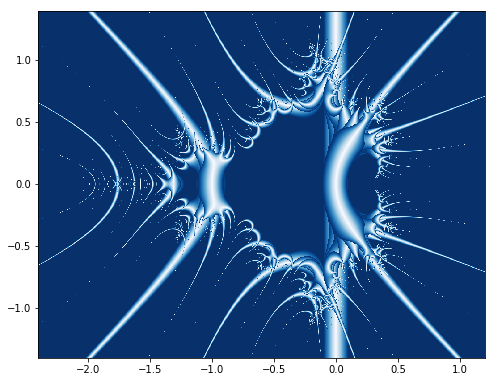Now we start seeing those 3D looking "objects" in the image.

The next function does the same orbit trapping but each iteration is assigned a different color. All the red "objects" come from points trapped during the first iteration, the green "objects" are trapped during the second iteration, and so on.

In :
def mandelbrottrapcolor(xrng, yrng, trapwidth=.4, colors=None):
''' Generate Mandelbrot using axis orbit trap colored by iteration.

Parameters
----------
xrng: array of real values defining width of plot
yrng: array of imag values defining height of plot
trapwidth: width of trap about real axis
colors: list of (r,g,b) tuples, one for each iteration.
'''
if colors is None:
# Colors as used by imshow are (r,g,b) tuples with each value in the range 0-1.
colors = [(1,0,0), (0,1,0), (1,0,1), (1,1,0), (0,1,1), (0,0,1)]

z0 = xrng + 1.0j * yrng.reshape(-1, 1)
z = np.zeros(z0.shape, dtype='complex')

# This time, image is full RGB data instead of just a single value for lookup table.
# Make an M x N x 3 array
image = np.zeros((len(xrng), len(yrng), 3))

# Loop through the different colors each iteration.
# Points trapped during each iteration will have their own color table, faded to black.
for r,g,b in colors:
z = z**2 + z0

# Find points that are within trapwidth of imaginary axis

# Use the distance from trap center to darken the color. Points on the axis
# will be full color value, points at trapwidth/2 will be reduced to (0, 0, 0) black.
distance = 1-abs(z[mask].real) / trapwidth*2  # Normalized distance from edge of trap

plt.figure(figsize=(8,8))
plt.imshow(image, aspect='equal', origin='lower',
extent=(xrng, xrng[-1], yrng, yrng[-1]))
return image

In :
image = mandelbrottrapcolor(np.linspace(-2.4,1.2,1000), np.linspace(-1.4,1.4,1000))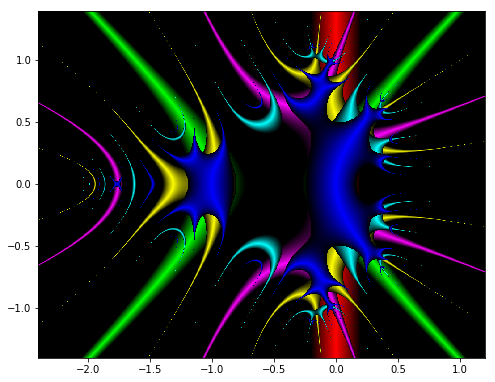#### IDEAS:¶

Create a copies of the mandelbrottrap or mandelbrottrapcolor function that:

• Traps along the real axis instead of imaginary axis
• Adds an offset to the axis trap. Instead of trapping when the point falls within $0 \pm 0.1$, try trapping the point when it hits $0.5 \pm 0.1$, etc.
• Trap using a circle, with color based on distance from the center of the circle.

## Saving Images¶

You could use matplotlib's savefig() function to save images, but it wants to save axes, titles, and so on. These can all be turned off, but it's easier to use a package that's meant for images. For that, there's Pillow (formerly called PIL in Python2).

We have two types of image data though. In the latest one we made an array of RGB values. These values should be saved directly to the output file. Except that Pillow wants RGB values as integers in the range 0-255, and matplotlib wanted them as floats from 0-1. So we have to scale first. And the origin is in the wrong place, so we need to flip the array (np.flipud).

In :
from PIL import Image   # In Python3, the package is called Pillow, but it's still imported as PIL.

image = mandelbrottrapcolor(np.linspace(-2.4,1.2,1000), np.linspace(-1.4,1.4,1000))  # Re-generate the image
im = Image.fromarray((np.flipud(image)*255).astype('uint8'), 'RGB')                  # Convert it to Pillow
im.save('orbitfractal.png')The earlier fractals (without orbit traps) we colored using matplotlib colormaps. The image data is just a float MxN array of escape times, not MxNx3 of actual RGB pixel data. We can use the colormap to convert it ourselves before saving. Note we also normalize the escape time to be in the 0-1 range before using the colormap.

In :
image_cmap = mandelbrot(np.linspace(-0.5,0,1000), np.linspace(-1.0,-0.5,1000), maxiter=50, cmap='viridis')  # Re-generate
image_cmap = np.flipud(image_cmap) / image_cmap.max()   # Normalize and flip
image_rgb = plt.get_cmap('viridis')(image_cmap, bytes=True)
im2 = Image.fromarray(image_rgb.astype('uint8'), 'RGBA')   # Convert to Pillow
im2.save('escapefractal.png')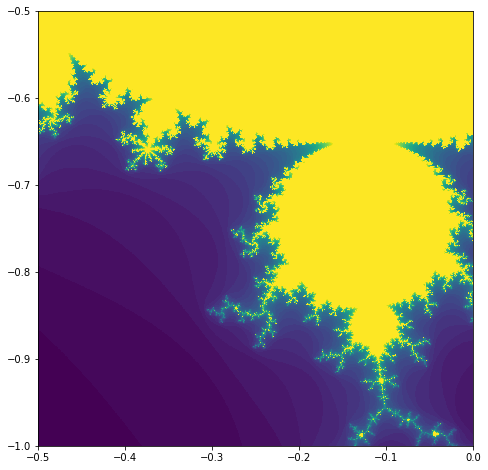### Oversampling¶

Open the saved image and zoom in to one of the thinner "dendrites". Notice how the lines start to look broken up into pixels. This can be fixed with oversampling and some image processing as a way to "antialias" the image. Generate the image twice as big as you want (here we render 2000x2000 pixels), and then reduce it by resampling (back down to 1000x1000) using Pillow. Of course it takes longer to generate 4 times as many pixels too. You won't see the results here in the notebook, but they're in the saved PNG image.

In :
image = mandelbrottrapcolor(np.linspace(-2.4,1.2,2000), np.linspace(-1.4,1.4,2000))  # Re-generate the image with 2x points in both dimensions
im = Image.fromarray((np.flipud(image)*255).astype('uint8'), 'RGB')
im.thumbnail((1000,1000))   # Reduce the size back down
im.save('orbitfractal_oversampled.png')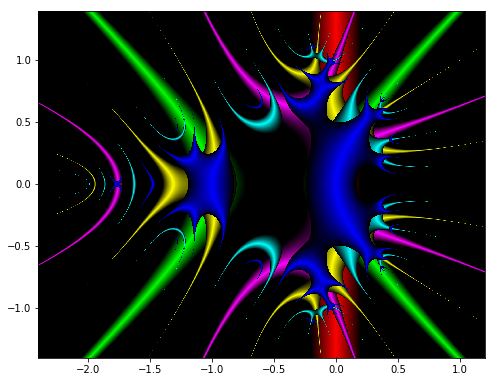## Math vs. Art¶

Now the art part of fractal images comes in. The knobs you can turn to generate different images include:

• The recurrence equation
• Location/zoom in the complex plane
• Location and shape of orbit traps
• Colors and coloring algorigthms
In [ ]:


In [ ]: